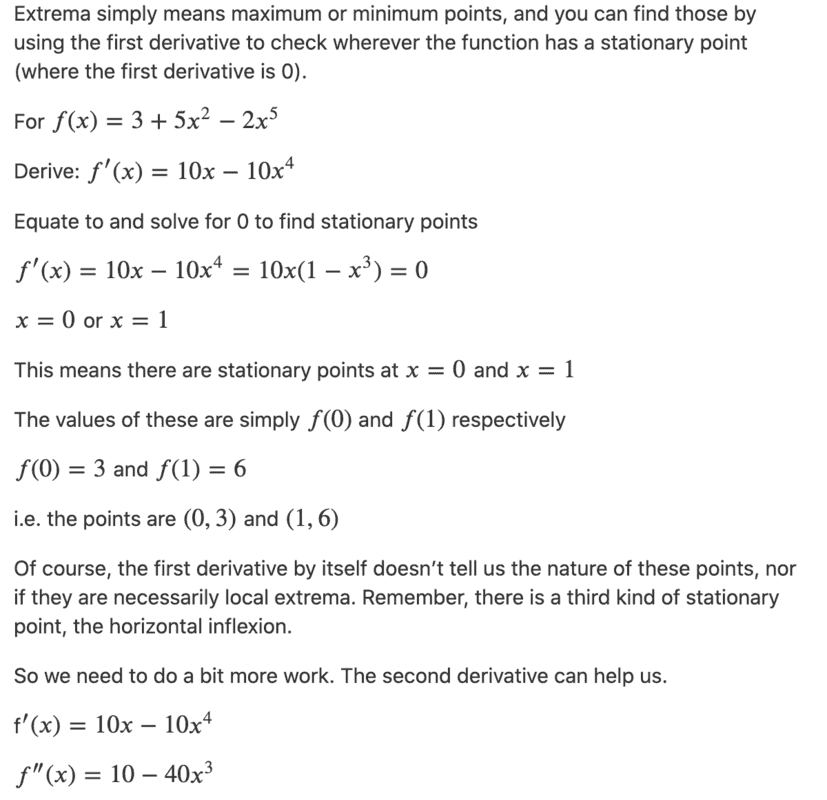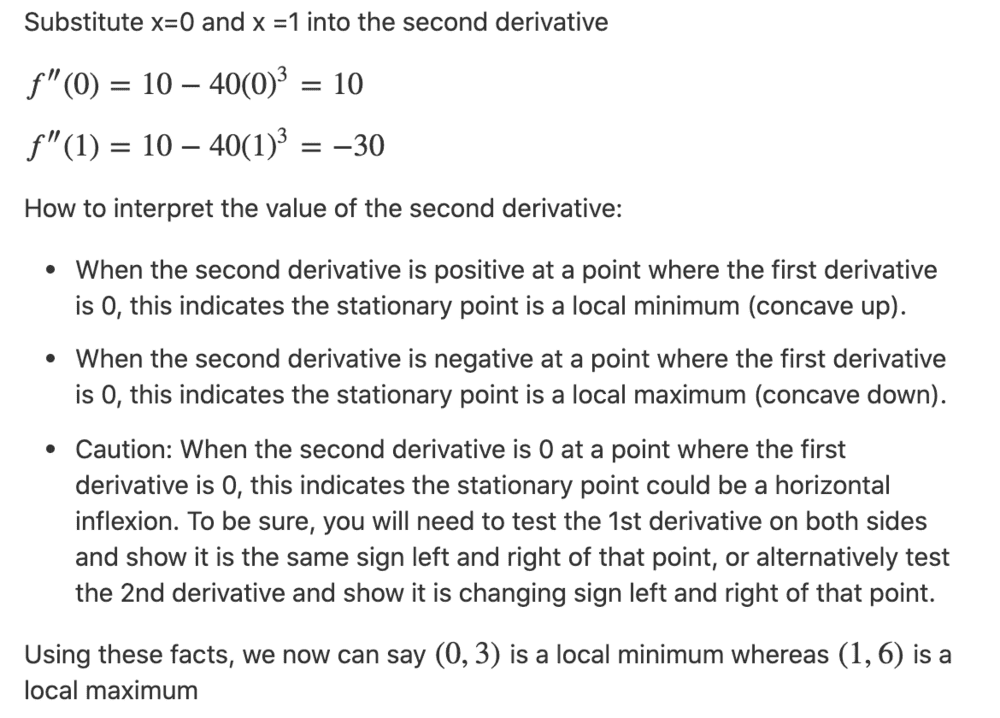# Find the local extrema then classify them

Homework Statement:
For the function ##f(x) = 3 +5x^2-2x^5##, find the local extrema. Then, classify the local extrema (maximum or minimum points) using the second derivative test.
Relevant Equations:
n/aSo this is a very long answer for a question worth 4 marks. Would it be a good idea to keep the explanation, or to have it strictly math only?

Last edited by a moderator:

etotheipi
So this is a very long answer for a question worth 4 marks. Would it be a good idea to keep the explanation, or to have it strictly math only?

How would you have answered the question differently, I wonder? You wouldn't need to write those last three bullet points, nor particularly any of the conversational text between the equations, but the key mathematical steps must be finding the turning points and classifying the turning points.

Last edited by a moderator:
How would you have answered the question differently? You wouldn't need to write those last three bullet points, nor particularly any of the conversational text between the equations, but the key mathematical steps must be finding the turning points and classifying the turning points.
Thank you for the suggestion